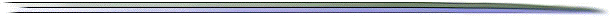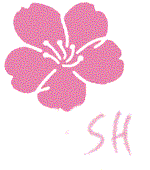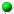# Response Surface Methodology

from the Perspective of Competitive Advantage

by
Edwin B. DeanMontgomery (1991) notes that
Response surface methodology, or RSM, is a collection of mathematical and statistical techniques that are useful for the modeling and analysis of problems in which a response of interest is influenced by several variables and the objective is to optimize the response. ... The process yield is a function of the levels ..., say

y = f(x1,x2) + e

where e represents the noise observed in the response y. If we denote the expected response by E(y) = f(x1,x2) = h, then the surface represented by

h = f(x1,x2)

is called the response surface.

In order to optimize competitiveness as a response, consider an objective of the form

y = av fv(x) + ac fc(x)

where av is a nonnegative number, ac is a nonpositive number, x is a vector of test variables, fv(x) is a function of positive qualities over the test variables, and fc(x) is a function of positive costs over the test variables. Then, maximizing y finds the most competitive set of test variables z(av,ac). The numbers av and ac are relative weights between qualty and cost. The trick is, of course, is to choose meaningful variables which drive quality and cost to find functions fv(x) and fc(x). Using response surface methodogy with an appropriate experimental design is an appropriate method of finding fv(x) and fc(x). Quality function deployment can be used to provide the quantities fv and parametric cost analysis can be used to provide the quantities fc.

Address information for a number of companies providing quality related software can be found in Struebing (1996).#### References

• Montgomery, D. C. (1991). Design and Analysis of Experiments, 3rd. ed., John Wiley and Sons, New York NY.
• Struebing, L. (1996). "Quality Progress' 13th Annual QA/QC Software Directory," Quality Progress, April, pp. 31-59#### BibliographiesResponse Surface MethodologyDesign of Experiments